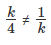# The equations kx + y - Quantitative Aptitude - Equation

## CAT 2020 - Slot 3 - Quantitative Aptitude - Equation - Question 15 - Let k be a constant. The equations kx + y

Q. 15: Let k be a constant. The equations kx + y = 3 and 4x + ky = 4 have a unique solution if and only if

##### 1. |k| = 22. k ≠ 23. |k| ≠ 24. k = 2

15. C.

Two generic equations, a1x + b1y + c1 = 0 and a2x + b2y + c2 = 0, will have a unique solution if and only if,  .

We have the equations kx + y = 3 and 4x + ky = 4,

For them to have unique solutions, they must satisfy the conditionk2 ≠ 4
k ≠ ±2
|k| ≠ 2

### Past Year Question Paper & SolutionsCounselling Session
By IIM Mentor

#### Free Material Area